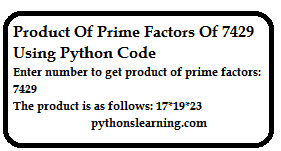Enter number to get product of prime factors ﻿

## product of prime factors of 7429 using python code

### Step for Calculate Product of Unique Prime Factors of a Number using python program

● The input is taken from the user.
● “CalculateProduct” function is called to find all unique prime factors and to display their product.
● Declare variable mult = 1 to store product of unique prime factors.
● Firstly, the number is checked to be divisible by 2.
● If the condition is satisfied then the variable is multiplied by 2.
● Then the number is divided by 2 recursively so as to remove 2 as a factor completely.
● Then the condition of prime factor is used. According to which the number will have prime factors up to its square root + 1 only and no more than that.
● So we declare variable “i” as 3.
● Increment it up to square root +1 of the number for our while loop.
● After which the number is checked to be divisible by all odd numbers i.e. 3 onwards (which is denoted by i).
● If the condition is satisfied then the variable is multiplied by i and the product is stored in the variable mult.
● Then the number is recursively divided by i so as to remove all the same factors.
● This makes the number uniquely divisible by the current value of i only.
● Thus the number would have all its prime factors product in mult.
● We have done operations on the number and if none of the conditions are satisfied then we check if it is greater than 2, if yes, then the number is prime. If not then the number is not prime and does not have any prime factor and print error.
● Print mult.

### python code for find Product Of Prime Factors Of 7429

``````INPUT :
import math

#Function to find product
def CalculateProduct(n):
mult = 1

#Check divisibility by 2 and multiply the variable with it if condition satisfied
if n % 2 == 0:
mult *= 2

#Recursively divide the number by 2 to remove it as a factor and make the product unique
while n % 2 == 0:
n = n / 2

i = 3
#Using prime factor condition(square root of number) and checking up to that point only
#as the number won't have any more prime factors further than that
while i < int(math.sqrt(n)+1):

#Check if number is divisible by the prime number and multiply the variable by it if condition satisfied
if n % i == 0:
mult *= i

#Divide the number recursively to make it uniquely divisible by that number
while n % i == 0:
n = n / i
i = i + 2

#Contition to see if the number itself is prime and multiply by initial value
if n > 2:
mult *= n

#Print the product
print("The product is as follows: ",mult)

#Take input from user
num = int(input("Enter number to get product of prime factors: "))

#Call function to find the product
CalculateProduct(num)
``````

OUTPUT :

Enter number to get product of prime factors: 150

The product is as follows:  30

i.e. The prime factors of the number 7429 is 17, 19, and 23.

Summary :

Kunal Goel